# Boundary conditions in Montjoie

The boundary conditions implemented in Montjoie are listed in the class BoundaryConditionEnum :

The boundary conditions are usually taken into account in the finite element matrix (through the variational formulation). Dirichlet and Supported boundary conditions are set by setting the concerned rows and columns to 0, with 1 on the diagonal. For inhomogenous Dirichlet, the columns are kept before erasing them so that the right hand side is modified. Periodic boundary conditions can be set by changing the numbers of degrees of freedom. Quasi-periodic boundary conditions are set either in the variational formulation or by modifying some rows of the finite element matrix.

The treatment of usual boundary conditions is performed in the class VarBoundaryCondition which is a base class of EllipticProblem.

## Public attributes of VarBoundaryCondition

 NewColumnNumbers_Impedance new column numbers when adding the surface integrals against basis functions ProcColumnNumbers_Impedance processor numbers (for columns) when adding the surface integrals against basis functions NewRowNumbers_Impedance new row numbers when adding the surface integrals against basis functions ProcRowNumbers_Impedance processor numbers (for rows) when adding the surface integrals against basis functions take_into_account_curvature_for_abc if true, the curvature is taken into account for absorbing boundary condition grazing_abc if true, the absorbing boundary condition is changed to handle mainly grazing waves gamma_cla_coef Parameter of the absorbing absorbing condition (for Helmholtz only) theta_cla_coef Parameter of the absorbing absorbing condition (for Helmholtz only) zeta_cla_coef Parameter of the absorbing absorbing condition (for Helmholtz only)

## Methods for ImpedanceFunction_Base

The class ImpedanceFunction_Base is the base class for all impedance classes (such as ImpedanceGeneric, ImpedanceABC or ImpedanceHighConductivity). These classes are used for impedance boundary conditions when the function AddMatrixImpedanceBoundary is called to add the corresponding terms to the finite element matrix.

 InvolveOnlyTangentialDofs returns true if only tangential dofs (associated with the surface) are involved in surface integrals SetCoefficient sets the impedance coefficients (in the case of an uniform impedance) GetCoefficient returns the impedance coefficient at a given quadrature point PresenceGradient returns true if the boundary integrals involve the gradient of basis functions EvaluateImpedancePhi evaluates the impedance involved in surface integrals against basis functions EvaluateImpedanceGrad evaluates the impedance involved in surface integrals against gradient of basis functions ApplyImpedancePhi_H1 applies the impedance involved in surface integrals against scalar basis functions ApplyImpedanceGrad applies the impedance involved in surface integrals against gradient of scalar basis functions ApplyImpedancePhi_Hcurl applies the impedance involved in surface integrals against basis functions (edge elements) ApplyImpedanceCurl applies the impedance involved in surface integrals against the curl of basis functions (edge elements) ApplyImpedancePhi_Hdiv applies the impedance involved in surface integrals against basis functions (facet elements) ApplyImpedanceDiv applies the impedance involved in surface integrals against the divergence of basis functions (facet elements)

## Transparent conditions

The details of transparent conditions is detailed in the thesis of M. Duruflé. The cornerstone of this method is a representation integral of the field outside a surface. In the case of Maxwell's equations, these formulas (known as Stratton-Chu formulas) are equal to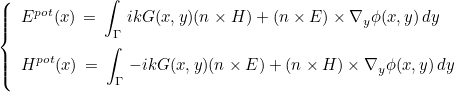with the Green kernel and dyadic Green function :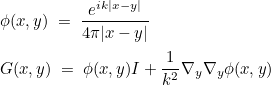There are similar expression for Helmholtz equation. Transparent conditions are not implemented with other equations. Γ is an intermediary surface surrounding the scatterer and Σ the external boundary. The boundary condition is then set on Σ to :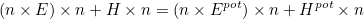The linear system to solve is then equal to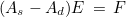The matrix As is the finite element matrix with a first-order absorbing boundary condition (this matrix is sparse) whereas the matrix Ad is the matrix containing the integral equation terms (this matrix has an important dense block). The matrix As is factorized (or solved iteratively), and the transparent condition is solved iteratively on the following linear system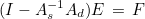If the distance between Γ and Σ is large enough (one wavelength is fine), the number of iterations needed to solve this system is quite small. The treatment of transparent conditions is handled by the class TransparencySolver.

### Public methods of TransparencySolver

 constructor of TransparencySolver UseTransparentCondition returns true if a transparent condition is set Solve solves the linear system associated with the transparent condition Init initialisation and computation of arrays needed for the resolution of the linear system associated with the transparent condition ComputeSolution computes the solution of As x = y ComputeGreenKernel computation of Green kernel and dyadic Green function ComputeSurfaceGammaAndAbsorbing computes the quadrature points on the two surfaces ComputeRightHandSide computes the matrix-vector product Ad x ComputeAndStoreEnPot computes the integral representation of E x n (and H x n) on all quadrature points GetSource computes the source term (on a given quadrature point) for the transparent condition ComputeIntegralRepresentation computes the integral representation of E x n (and H x n) on a given point

For Helmholtz and Maxwell's equations, it is also possible to compute the far field (through an integral over a surface). The computation of the far field (also known as radar cross section) is performed in class VarComputationRCS

### Public methods of VarComputationRCS

 RcsToBeComputed returns true if a radar cross section needs to be computed GetNbAngles returns the number of angles for which we want to know the far field GetNbPointsOutside returns the number of points for which we want to know the field GetInterpolationMesh returns the surface mesh used for the computation of the RCS GetRcsType returns the type of radar cross section (monostatic or bistatic) GetOutsidePoint returns the coordinates of the points where we want to know the field SetOutsidePoints sets the coordinates of the points where we want to know the field SetTimeStep sets the time step (for unsteady equations) InitComputationRCS initializes the object before computing RCS ComputeIntegralRepresentation computes the value of the field outside the computational domain with an integral representation ComputeRCS effective computation of the radar cross section

#### Dirichlet Boundary condition (LINE_DIRICHLET)

Dirichlet boundary conditions can be homogeneous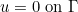or inhomogeneousIn the case of edge elements, Dirichlet condition is actually a perfectly conductor condition :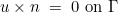Dirichlet condition is set by setting to 0 the concerned rows and columns and putting 1 on the diagonal. This coefficient one can be changed for the computation of eigenvalues to avoid the pollution of the spectrum with eigenvalues equal to 1. For discontinuous Galerkin formulations, the Dirichlet condition is handled in the variational formulation.

Neumann boundary conditions are equal for the Laplace and Helmholtz equation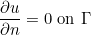In the case of Maxwell's equations, it refers to :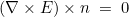In the case of elastodynamics, it refers to :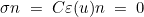In the case of aeroacoustics, it refers to :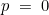As you can see, this boundary condition depends on the considered equation, but usually is associated with the boundary condition that will cancel the boundary integral coming from the integration by parts.

#### Supported Boundary condition (LINE_SUPPORTED)

Supported boundary conditions consists of imposing a Dirichlet condition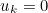for only a subset of components k of the unknown u. The components are given by inserting a line SupportedComponents = 1 3 in the datafile. For other components, a natural condition is imposed (no boundary terms like for Neumann condition).

#### Dirichlet to Neumann (LINE_DTN)

This condition is reserved for a future implementation of Dirichlet-to-Neumann operators in Montjoie

#### Transmission conditions (LINE_TRANSMISSION)

Transmission conditions are present for some models in Montjoie. The documentation will be updated later.

#### False boundary condition (LINE_NEIGHBOR)

LINE_NEIGHBOR is used for boundaries located at the interface between two processor. For such boundaries, no boundary condition is needed. However in Montjoie, since the face is isolated (no adjacent face in the current processor), a boundary condition is needed. That's why, such faces are affected with this false boundary condition.

#### Thin-slot models (LINE_THIN_SLOT)

Thin slot models are implemented in Montjoie for 2-D Helmholtz equation. The documentation will be updated later.

#### High-conductivity models (LINE_HIGH_CONDUCTIVITY)

High-conductivity models are implemented in Montjoie for Helmholtz and Maxwell's equations. The documentation will be updated later.

#### Robin boundary conditions (LINE_IMPEDANCE)

Robin boundary conditions consists of considering.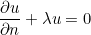for scalar equations. The documentation will be updated later.

#### Absorbing boundary conditions (LINE_ABSORBING)

Absorbing boundary conditions are used to truncate the computational domain. For most of equations, only first-order absorbing boundary conditions are implemented. For Helmholtz equations, it consists of considering.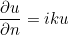where k is the wave number.

### NewColumnNumbers_Impedance, ProcColumnNumbers_Impedance

This attribute is an array used when adding boundary terms in the finite element matrix. You can modify the column numbers of the degrees of freedom such that the term.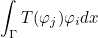is added to the entry i, NewColumnNumbers_Impedance(j) of the matrix (instead of i, j). ProcColumnNumbers_Impedance is used in parallel to store the processor associated with the new number. If the processor is equal to the current processor, the column number is local, whereas it is global for a different processor.

#### Example :

    EllipticProblem<LaplaceEquation<Dimension2> > var;;

// constructing the problem (mesh, finite element)
All_LinearSolver* solver;

// initializing an empty matrix;
int N = var.GetNbDof();
DistributedMatrix<Real_wp, General, ArrayRowSparse> A;
A.Reallocate(N, N);

// filling the new column numbers (here we place random numbers)
var.NewColumnNumbers_Impedance.Reallocate(N);
var.NewColumnNumbers_Impedance.FillRand();

// for parallel computation you can also set a different processor number
var.ProcColumnNumbers_Impedance.Reallocate(N);
var.ProcColumnNumbers_Impedance.Fill(0);

// then you can add the impedance term
GlobalGenericMatrix<Real_wp> nat_mat;
IVect ref(var.mesh.GetNbReferences()+1); ref.Zero(); ref(3) = 1;
var.AddMatrixImpedanceBoundary(1.0, ref, 1, nat_mat, A, 0, 0, imped, true, false, var);



### NewRowNumbers_Impedance, ProcRowNumbers_Impedance

This attribute is an array used when adding boundary terms in the finite element matrix. You can modify the row numbers of the degrees of freedom such that the term.is added to the entry NewRowNumbers_Impedance(i), j of the matrix (instead of i, j). ProcRowNumbers_Impedance is used in parallel to store the processor associated with the new number. If the processor is equal to the current processor, the row number is local, whereas it is global for a different processor.

#### Example :

    EllipticProblem<LaplaceEquation<Dimension2> > var;;

// constructing the problem (mesh, finite element)
All_LinearSolver* solver;

// initializing an empty matrix;
int N = var.GetNbDof();
DistributedMatrix<Real_wp, General, ArrayRowSparse> A;
A.Reallocate(N, N);

// filling the new row numbers (here we place random numbers)
var.NewRowNumbers_Impedance.Reallocate(N);
var.NewRowNumbers_Impedance.FillRand();

// for parallel computation you can also set a different processor number
var.ProcRowNumbers_Impedance.Reallocate(N);
var.ProcRowNumbers_Impedance.Fill(0);

// then you can add the impedance term
GlobalGenericMatrix<Real_wp> nat_mat;
IVect ref(var.mesh.GetNbReferences()+1); ref.Zero(); ref(3) = 1;
var.AddMatrixImpedanceBoundary(1.0, ref, 1, nat_mat, A, 0, 0, imped, false, true, var);



### take_into_account_curvature_for_abc

This attribute is a boolean. If it is set to true, the absorbing boundary condition will include the term due to the curvature (1/R term). This feature is only implemented for Helmholtz equation. It is usually modified by inserting a line ModifiedImpedance = CURVE in the data file.

### grazing_abc

This attribute is a boolean. If it is set to true, the absorbing boundary condition will be modified to handle correctly grazing waves. This feature is only implemented for Helmholtz equation. It is usually modified by inserting a line OrderAbsorbingBoundaryCondition = 1 GRAZING in the data file.

### gamma_cla_coef, theta_cla_coef, zeta_cla_coef

These attributes are doubles that are used to parametrize absorbing boundary conditions. This feature is only implemented for Helmholtz equation. It is usually modified by inserting a line OrderAbsorbingBoundaryCondition = 2 PARAMETERS 0.2 0.5 1.0 in the data file.

### GetInitialSymmetrization

This method returns true if the matrix (for a mixed formulation) can be symmetrized (with respect to boundary conditions). It does not mean that the matrix will be symmetrized when PerformFactorizationStep is called.

#### Example :

    EllipticProblem<LaplaceEquation<Dimension2> > var;;

// constructing the problem (mesh, finite element)
All_LinearSolver* solver;

// the matrix can be symmetrized ?
bool init_sym = var.GetInitialSymmetrization();


### GetBoundaryConditionId

#### Syntax

 int GetBoundaryConditionId(IVect ref, int pos, VectString parameters, bool& periodic)

This method returns the integer corresponding to the boundary condition given as a string (or a list of strings). Usually, only parameters(pos) is used to determine the boundary condition. For more complex boundary conditions, several parameters can be used (parameters(pos), parameters(pos+1), etc). The first argument is not used, the last argument is true if the boundary condition corresponds to a periodic (or quasi-periodic condition).

#### Example :

    EllipticProblem<LaplaceEquation<Dimension2> > var;;

// constructing the problem (mesh, finite element)
All_LinearSolver* solver;

// boundary condition id (e.g. BoundaryConditionEnum::LINE_DIRICHLET) ?
IVect ref; int pos = 1; VectString parameters(pos+1);
parameters(pos) = "DIRICHLET"; bool periodic;
int id = var.GetBoundaryConditionId(ref, pos, parameters, periodic);


### GetNbDirichletDof

#### Syntax

 int GetNbDirichletDof() const

This method returns the number of degrees of freedom associated with a Dirichlet condition (u = f). Only degrees of freedom of the current processor are counted.

#### Example :

    EllipticProblem<LaplaceEquation<Dimension2> > var;;

// constructing the problem (mesh, finite element)
All_LinearSolver* solver;

// number of Dirichlet dofs for the current processor
nb_dir_dof = var.GetNbDirichletDof();


### GetNbGlobalDirichletDof

#### Syntax

 int GetNbGlobalDirichletDof() const

This method returns the number of degrees of freedom associated with a Dirichlet condition (u = f). Degrees of freedom of all the processors are counted.

#### Example :

    EllipticProblem<LaplaceEquation<Dimension2> > var;;

// constructing the problem (mesh, finite element)
All_LinearSolver* solver;

// number of Dirichlet dofs for the overall simulation (with all processors)
nb_dir_dof = var.GetNbGlobalDirichletDof();


### GetDirichletDofNumber

#### Syntax

 int GetDirichletDofNumber(int i ) const const IVect& GetDirichletDofNumber() const

This method returns the dof number of the i-th Dirichlet dof. In the second syntax, you can retrieve the array containing Dirichlet dofs.

#### Example :

    EllipticProblem<LaplaceEquation<Dimension2> > var;;

// constructing the problem (mesh, finite element)
All_LinearSolver* solver;

// number of Dirichlet dofs for the current processor
nb_dir_dof = var.GetNbGlobalDirichletDof();

// loop over Dirichlet dofs
for (int i = 0; i < nb_dir_dof; i++)
{
// you can retrieve a single dof
int num_dof = var.GetDirichetDofNumber(i);
}

// or all the dofs
const IVect& dir_dof = var.GetDirichletDofNumber();


### IsDofDirichlet

#### Syntax

 bool IsDofDirichlet(int i ) const

This method returns true if the degree of freedom i is associated with a Dirichlet condition (u = f).

#### Example :

    EllipticProblem<LaplaceEquation<Dimension2> > var;;

// constructing the problem (mesh, finite element)
All_LinearSolver* solver;

// dof 32 is Dirichlet ?
bool dof_dir = var.IsDofDirichlet(32);


### GetIsDofDirichlet

#### Syntax

 const Vector& GetIsDofDirichlet() const

This method returns the array containing booleans to know if a degree of freedom is Dirichlet or not.

#### Example :

    EllipticProblem<LaplaceEquation<Dimension2> > var;;

// constructing the problem (mesh, finite element)
All_LinearSolver* solver;

// dof 32 is Dirichlet ?
bool dof_dir = var.IsDofDirichlet(32);

// if you want all the values at once
const VectBool& arr_dir = var.GetIsDofDirichlet();


### UseSymmetricDirichlet

#### Syntax

 bool UseSymmetricDirichlet() const

This method returns true if rows and columns associated with Dirichlet dofs are removed (and a non-null coefficient is put on the diagonal). This treatment is already performed for symmetric matrices. If the method returns true, the treatment will also be performed for unsymmetric matrices. If the method returns false, only rows are erased for unsymmetric matrices.

#### Example :

    EllipticProblem<LaplaceEquation<Dimension2> > var;;

// constructing the problem (mesh, finite element)
All_LinearSolver* solver;

// rows and columns are eliminated for Dirichlet ?
bool dir_sym = var.UseSymmetricDirichlet();


### EnableSymmetricDirichlet

#### Syntax

 bool EnableSymmetricDirichlet(bool sym = true) const

If the argument sym is true, rows and columns associated with Dirichlet dofs are removed (and a non-null coefficient is put on the diagonal). This treatment is already performed for symmetric matrices, but will be also performed for unsymmetric matrices. The aim is to obtain a better conditioning of the finite element matrix. This feature is usually activated by inserting a line ForceDirichletSymmetry = YES in the datafile.

#### Example :

    EllipticProblem<LaplaceEquation<Dimension2> > var;;

// constructing the problem (mesh, finite element)
All_LinearSolver* solver;

// if we want to eliminate rows and columns for Dirichlet dofs
var.EnableSymmetricDirichlet();


### GetCoefficientDirichlet

#### Syntax

 Real_wp GetCoefficientDirichlet() const

This method returns the coefficient set on the diagonal for Dirichlet dofs. Rows associated with Dirichlet dofs are removed, only a coefficient is kept on the diagonal.

#### Example :

    EllipticProblem<LaplaceEquation<Dimension2> > var;;

// constructing the problem (mesh, finite element)
All_LinearSolver* solver;

// coefficient on diagonal for Dirichlet ?
Real_wp coef = var.GetCoefficientDirichlet();


### SetCoefficientDirichlet

#### Syntax

 void SetCoefficientDirichlet(Real_wp coef) const

This method sets the coefficient set on the diagonal for Dirichlet dofs. Rows associated with Dirichlet dofs are removed, only a coefficient is kept on the diagonal. You can also modify this coefficient (initially equal to one) by inserting a line DirichletCoefMatrix = 2.0 in the datafile.

#### Example :

    EllipticProblem<LaplaceEquation<Dimension2> > var;;

// constructing the problem (mesh, finite element)
All_LinearSolver* solver;

// modifying coefficient on diagonal for Dirichlet
var.SetCoefficientDirichlet(Real_wp(2));


### SetDirichletDof

#### Syntax

 void SetDirichletDof(int n, bool b) const

This method sets the n-th degree of freedom as a Dirichlet dof (if b is true). Once you have manually modified Dirichlet dofs, you need to call the method UpdateDirichletDofs to reconstruct the array storing the Dirichlet dofs.

#### Example :

    EllipticProblem<LaplaceEquation<Dimension2> > var;;

// constructing the problem (mesh, finite element)
All_LinearSolver* solver;

// manually adding Dirichlet dofs (not recommended)
var.SetDirichletDof(3, true);
var.SetDirichletDof(12, true);

// you can also remove Dirichlet dofs
var.SetDirichletDof(11, false);

// once you finished, you call UpdateDirichletDofs
var.UpdateDirichletDofs();


### GetNbSupportedComponents

#### Syntax

 int GetNbSupportedComponents() const

This method returns the number of components for the supported boundary condition.

#### Example :

    EllipticProblem<LaplaceEquation<Dimension2> > var;;

// constructing the problem (mesh, finite element)
All_LinearSolver* solver;

int nb_comp = var.GetNbSupportedComponents();
// loop over supported components
for (int k = 0; k < nb_comp; k++)
{
// displaying the list of components for the supported boundary condition
cout << "component " << var.GetSupportedComponent(k) << endl;
}


### GetSupportedComponent

#### Syntax

 int GetSupportedComponent(int k ) const

This method returns a component number for the supported boundary condition. The supported boundary condition consists of imposing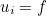for integers i that corresponds to component numbers.

#### Example :

    EllipticProblem<LaplaceEquation<Dimension2> > var;;

// constructing the problem (mesh, finite element)
All_LinearSolver* solver;

int nb_comp = var.GetNbSupportedComponents();
// loop over supported components
for (int k = 0; k < nb_comp; k++)
{
int i = var.GetSupportedComponent(k);
// displaying the list of components for the supported boundary condition
cout << "component " << i << endl;
// we have u_i = f
}


### SetSupportedComponents

#### Syntax

 void SetSupportedComponents(IVect num ) const

This method sets the list of component numbers for the supported boundary condition. The supported boundary condition consists of imposingfor integers i that corresponds to component numbers. Usually the components of the supported boundary condition are set by inserting a line SupportedComponents = 2 5 in the datafile.

#### Example :

    EllipticProblem<LaplaceEquation<Dimension2> > var;;

// constructing the problem (mesh, finite element)
All_LinearSolver* solver;

// setting manually components for the supported boundary condition
IVect num(2); num(0) = 2; num(1) = 5;
var.SetSupportedComponents(num);


### ImposeNullDirichletCondition

#### Syntax

 void ImposeNullDirichletCondition(Vector& u) const

This method enforces an homogeneous Dirichlet conditionfor the input vector u.

#### Example :

    EllipticProblem<LaplaceEquation<Dimension2> > var;;

// constructing the problem (mesh, finite element)
All_LinearSolver* solver;

VectReal_wp u(var.GetNbDof()); u.FillRand();

// setting u = 0 for Dirichlet dofs
var.ImposeNullDirichletCondition(u);


### GetHighConductivityOrder

#### Syntax

 int GetHighConductivityOrder() const

This method returns the order of approximation for the high-conductivity boundary condition.

#### Example :

    EllipticProblem<LaplaceEquation<Dimension2> > var;;

// constructing the problem (mesh, finite element)
All_LinearSolver* solver;

// order for high-conductivity boundary condition
int r_conduc = var.GetHighConductivityOrder();


### FindDofsOnReference

#### Syntax

 void FindDofsOnReference(const VarProblem& var, const IVect& ref_cond, int ref_target, IVect& Dofs) const

This method retrieves the degrees of freedom associated with faces (edges in 2-D) such that the reference ref satisfies ref_cond(ref) = ref_target.

#### Parameters

var (in)
instance of VarProblem
ref_cond (in)
references i such that ref_cond(i) = ref_target are considered
ref_target (in)
target reference
Dofs (out)
list of degrees of freedom located on the selected referenced faces

#### Example :

    EllipticProblem<LaplaceEquation<Dimension2> > var;;

// constructing the problem (mesh, finite element)
All_LinearSolver* solver;

// degrees of freedom for reference 2 and 3 ?
IVect ref_cond(var.mesh.GetNbReferences()+1); ref_cond.Zero();
int ref_target = 1; ref_cond(2) = ref_target; ref_cond(3) = ref_target;

IVect Dofs;
var.FindDofsOnReference(var, ref_cond, ref_target, Dofs);


### TreatDirichletCondition

#### Syntax

 void TreatDirichletCondition()

This method computes all the degrees of freedom located on Dirichlet boundaries. This method does not need to be called in a regular use, since it is already called by ComputeMeshAndFiniteElement.

### SetDirichletDofs

#### Syntax

 void SetDirichletDofs(int N, IVect dof_list)

This method sets all the degrees of freedom considered as Dirichlet dofs (i.e. such that the equation u = f is imposed on these dofs). Previous Dirichlet dofs are removed and replaced by the provided list. After calling this method, you do not need to call UpdatedirichletDofs.

#### Parameters

N (in)
number of Dirichlet dofs
dof_list (in)
dof numbers

#### Example :

    EllipticProblem<LaplaceEquation<Dimension2> > var;;

// constructing the problem (mesh, finite element)
All_LinearSolver* solver;

// degrees of freedom for reference 2 and 3 ?
IVect ref_cond(var.mesh.GetNbReferences()+1); ref_cond.Zero();
int ref_target = 1; ref_cond(2) = ref_target; ref_cond(3) = ref_target;

IVect Dofs;
var.FindDofsOnReference(var, ref_cond, ref_target, Dofs);

// then you can choose to impose Dirichlet on these dofs (and remove previous Dirichlet dofs)
var.SetDirichletDofs(Dofs.GetM(), Dofs);



### ResizeNbDof

#### Syntax

 void ResizeNbDof(int N)

This method changes the number of degrees of freedom of the global problem. It is better to call that method other SetNbDof since IsDofDirichlet will work correctly.

#### Example :

    EllipticProblem<LaplaceEquation<Dimension2> > var;;

// constructing the problem (mesh, finite element)
All_LinearSolver* solver;

// you want to add a new degree of freedom
int N = var.GetNbDof();
var.ResizeNbDof(N+1);



### ComputeDirichletCoef

#### Syntax

 void ComputeDirichletCoef(VirtualMatrix& A)

This method evaluates the largest eigenvalue of the input matrix A (by iterative power method) and returns twice this estimation. This value can then be used as Dirichlet coefficient (on the diagonal), such that eigenvalues due to Dirichlet condition will be outside the spectrum of interest.

#### Example :

    EllipticProblem<LaplaceEquation<Dimension2> > var;;

// constructing the problem (mesh, finite element)
All_LinearSolver* solver;

// computing the matrix
GlobalGenericMatrix<Real_wp> nat_mat;
DistributedMatrix<Real_wp, Symmetric, ArrayRowSymSparse> A;

// coefficient for Dirichlet diagonal ?
Real_wp coef = var.ComputeDirichletCoef(A);


### UpdateDirichletDofs

#### Syntax

 void UpdateDirichletDofs()

This method updates the arrays for Dirichlet dofs. It has to be called if Dirichlet dofs have been set manually with SetDirichletDof.

#### Example :

    EllipticProblem<LaplaceEquation<Dimension2> > var;;

// constructing the problem (mesh, finite element)
All_LinearSolver* solver;

// manually adding Dirichlet dofs (not recommended)
var.SetDirichletDof(3, true);
var.SetDirichletDof(12, true);

// you can also remove Dirichlet dofs
var.SetDirichletDof(11, false);

// once you finished, you call UpdateDirichletDofs
var.UpdateDirichletDofs();


### ApplyDirichletCondition

#### Syntax

 void ApplyDirichletCondition(SeldonTranspose trans , FemMatrixFreeClass& , A, Vector& b , int k = 0) const

This method modifies the right hand side because of inhomogeneous Dirichlet condition. To recover the symmetry of the matrix, rows and columns associated with Dirichlet dofs are removed. As a result, the right hand side must be modified to take into account inhomogeneous Dirichlet condition (u = f). On input, the right hand side b contains values of f on degrees of freedom. This method does not need to be called in a regular use, since ComputeSolution already calls it.

#### Parameters

trans (in)
Do we want to solve A x = b or the transpose system ?
A (in)
iterative finite element matrix
b (inout)
right hand side to modify
k (optional)
right hand side number

### GetNbModes

#### Syntax

 int GetNbModes() const

This method returns the number of modes to compute in order to recover the solution for a source that has no symmetry (only the computational domain has symmetry). For example, in case of cyclic domains, it is the number of Fourier modes. If the computational domain has no symmetry, it returns one.

#### Example :

    EllipticProblem<LaplaceEquation<Dimension2> > var;;

// constructing the problem (mesh, finite element)
All_LinearSolver* solver;

// number of modes to compute the solution (for periodic/cyclic domains)
nb_modes = var.GetNbModes();


### GetNbModesSource

#### Syntax

 int GetNbModesSource() const

This method returns the number of modes to compute in order to recover the solution for a source that has no symmetry (only the computational domain has symmetry). For example, in case of cyclic domains, it is the number of Fourier modes. If the computational domain has no symmetry, it returns one. The difference between GetNbModes and GetNbModesSource happens for axisymmetric computation. In that case, each mode is solved independently, and there is only "one mode" for the computation of the source, since we compute directly the decomposition of the source on the required mode.

#### Example :

    EllipticProblem<LaplaceEquation<Dimension2> > var;;

// constructing the problem (mesh, finite element)
All_LinearSolver* solver;

// number of modes for the evaluation of the source
nb_modes = var.GetNbModesSource();


### GetModeNumber

#### Syntax

 int GetModeNumber(int i ) const

This method returns the mode number of the i-th mode to compute. For instance, if we consider axisymmetric computations, where the solution is written as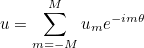If all the modes (between -M and M) are involved, the first computed mode will be m=0, then m=1, then m=-1, etc. So the method GetModeNumber will return -1 for i = 2.

#### Example :

    EllipticProblem<LaplaceEquation<Dimension2> > var;;

// constructing the problem (mesh, finite element)
All_LinearSolver* solver;

// number of modes for the evaluation of the source
nb_modes = var.GetNbModesSource();

// first mode to be computed
int m = var.GetModeNumber(0);


### GetCurrentModeNumber

#### Syntax

 int GetCurrentModeNumber() const

This method returns the mode number of the current mode that is solved.

#### Example :

    EllipticProblem<HelmholtzEquationAxi> var;;

// constructing the problem (mesh, finite element)
All_LinearSolver* solver;

// setting a mode number (value of m)
var.SetCurrentModeNumber(1);

// then you can retrieve this number
int m = var.GetCurrentModeNumber();


### SetCurrentModeNumber

#### Syntax

 void SetCurrentModeNumber(int m ) const

This method sets the mode number of the current mode that will be solved.

#### Example :

    EllipticProblem<HelmholtzEquationAxi> var;;

// constructing the problem (mesh, finite element)
All_LinearSolver* solver;

// setting a mode number (value of m)
var.SetCurrentModeNumber(1);

// then you can retrieve this number
int m = var.GetCurrentModeNumber();


### ModesNotStored

#### Syntax

 bool ModesNotStored() const

This method returns true if the modes are not stored. When the modes are not stored, the final solution is modified at each computation. If the modes are stored, fft can be used to obtain quickly the final solution. This functionality is turned on/off by inserting a line StorageModes = YES in the data file.

#### Example :

    EllipticProblem<LaplaceEquation<Dimension2> > var;;

// constructing the problem (mesh, finite element)
All_LinearSolver* solver;

// modes will be stored ?
bool store_modes = var.ModesNotStored();


### ForceStorageModes

#### Syntax

 bool ForceStorageModes(bool store = true)

This method enables (or disables) the storage of modes. When the modes are not stored, the final solution is modified at each computation. If the modes are stored, fft can be used to obtain quickly the final solution. This functionality is usually turned on/off by inserting a line StorageModes = YES in the data file.

#### Example :

    EllipticProblem<LaplaceEquation<Dimension2> > var;;

// constructing the problem (mesh, finite element)
All_LinearSolver* solver;

// if you want to force the storage of modes
var.ForceStorageModes(true);


### GetSymmetryType

#### Syntax

 int GetSymmetryType()

This method returns the type of symmetry that will be used for the computation of the solution. It can be equal to

• NO_SYMMETRY : no symmetry is present (default case) : only one mode is computed
• PERIODIC_THETA : cyclic domain (only a section is meshed)
• PERIODIC_X : periodic domain in x (only a cell is meshed)
• PERIODIC_Y : periodic domain in y (only a cell is meshed)
• PERIODIC_Z : periodic domain in z (only a cell is meshed)
• PERIODIC_XY : periodic domain in x and y (only a cell is meshed)
• PERIODIC_XZ : periodic domain in x and z(only a cell is meshed)
• PERIODIC_YZ : periodic domain in y and z (only a cell is meshed)
• PERIODIC_XYZ : periodic domain in x, y and z (only a cell is meshed)
• PERIODIC_ZTHETA : periodic domain in z and theta (only a cell is meshed)

The periodic conditions are set when inserting a line ConditionReference = 1 2 CYCLIC in the data file.

#### Example :

    EllipticProblem<LaplaceEquation<Dimension2> > var;;

// constructing the problem (mesh, finite element)
All_LinearSolver* solver;

// type of periodicity in the mesh ?
int sym = var.GetSymmetryType();


### GetNbPeriodicDof

#### Syntax

 int GetNbPeriodicDof()

This method returns the number of degrees of freedom that are labelled periodic. For these degrees of freedom, the following equation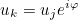replaces the variational formulation. This replacement is performed only for a strong formulation of quasi-periodic conditions (UseSameDofsForPeriodicCondition = NO in the data file).

#### Example :

    EllipticProblem<LaplaceEquation<Dimension2> > var;;

// constructing the problem (mesh, finite element)
All_LinearSolver* solver;

// number of dofs where quasi-periodic condition is applied
int nb_dof_per = var.GetNbPeriodicDof();


### GetPeriodicDof

#### Syntax

 int GetPeriodicDof(int i)

This method returns the number k of the i-th periodic dof. For these degrees of freedom, the following equationreplaces the variational formulation. This replacement is performed only for a strong formulation of quasi-periodic conditions (UseSameDofsForPeriodicCondition = NO in the data file).

#### Example :

    EllipticProblem<LaplaceEquation<Dimension2> > var;;

// constructing the problem (mesh, finite element)
All_LinearSolver* solver;

// number of dofs where quasi-periodic condition is applied
int nb_dof_per = var.GetNbPeriodicDof();

// loop over periodic dofs
for (int i = 0; i < nb_dof_per; i++)
{
// number of the periodic dof
int k = var.GetPeriodicDof(i);

// number of the original dof
int j = var.GetOriginalPeriodicDof(i);

// we have an equation u_k = u_j * e^{i phase}
}


### GetOriginalPeriodicDof

#### Syntax

 int GetOriginalPeriodicDof(int i)

This method returns the number j of the original degree of freedom associated with the i-th periodic dof. For periodic degrees of freedom, the following equationreplaces the variational formulation. This replacement is performed only for a strong formulation of quasi-periodic conditions (UseSameDofsForPeriodicCondition = NO in the data file).

#### Example :

    EllipticProblem<LaplaceEquation<Dimension2> > var;;

// constructing the problem (mesh, finite element)
All_LinearSolver* solver;

// number of dofs where quasi-periodic condition is applied
int nb_dof_per = var.GetNbPeriodicDof();

// loop over periodic dofs
for (int i = 0; i < nb_dof_per; i++)
{
// number of the periodic dof
int k = var.GetPeriodicDof(i);

// number of the original dof
int j = var.GetOriginalPeriodicDof(i);

// we have an equation u_k = u_j * e^{i phase}
}


### GetProcOriginalPeriodicDof

#### Syntax

 int GetProcOriginalPeriodicDof(int i)

This method returns the processor owning the original degree of freedom associated with the i-th periodic dof. For periodic degrees of freedom, the following equationreplaces the variational formulation. This replacement is performed only for a strong formulation of quasi-periodic conditions (UseSameDofsForPeriodicCondition = NO in the data file).

#### Example :

    EllipticProblem<LaplaceEquation<Dimension2> > var;;

// constructing the problem (mesh, finite element)
All_LinearSolver* solver;

// number of dofs where quasi-periodic condition is applied
int nb_dof_per = var.GetNbPeriodicDof();

// loop over periodic dofs
for (int i = 0; i < nb_dof_per; i++)
{
// number of the periodic dof
int k = var.GetPeriodicDof(i);

// number of the original dof
int j = var.GetOriginalPeriodicDof(i);

// processor that owns this dof
int proc = var.GetProcOriginalPeriodicDof(i);

// we have an equation u_k = u_j * e^{i phase}
}


### GetFormulationForPeriodicCondition

#### Syntax

 int GetFormulationForPeriodicCondition()

This method returns the formulation used to handle quasi-periodic conditions. The method is chosen by inserting a line UseSameDofsForPeriodicCondition = NO in the data file. It can be equal to :

• SAME_PERIODIC_DOFS : the original and periodic dof have the same number. This formulation is perfect for periodic conditions, but quasi-periodic conditions cannot be handled.
• STRONG_PERIODIC : the original and periodic dof have different numbers, an equation uk = uj ei φ is imposed strongly.
• WEAK_PERIODIC : the quasi-periodic condition is enforced weakly (with surface integrals).

#### Example :

    EllipticProblem<LaplaceEquation<Dimension2> > var;;

// constructing the problem (mesh, finite element)
All_LinearSolver* solver;

// number of dofs where quasi-periodic condition is applied
int nb_dof_per = var.GetNbPeriodicDof();

// formulation
int form = var.GetFormulationForPeriodicCondition();
// can be equal to MeshNumbering_Base<Real_wp>::STRONG_PERIODIC



### SetModesToCompute

#### Syntax

 void SetModesToCompute(IVect num )

This method sets the list of modes that need to be solved for the computation of the solution.

#### Example :

    EllipticProblem<LaplaceEquation<Dimension2> > var;;

// constructing the problem (mesh, finite element)
All_LinearSolver* solver;

// setting manually the list of modes
IVect num(2); num(0) = 1; num(1) = 3;
var.SetModesToCompute(num);

// GetModeNumber(0) will return 1
int m0 = var.GetModeNumber(0);


### PushBackMode

#### Syntax

 void PushBackMode(int n )

This method pushes a mode number at the end of the list of modes that need to be solved for the computation of the solution.

#### Example :

    EllipticProblem<LaplaceEquation<Dimension2> > var;;

// constructing the problem (mesh, finite element)
All_LinearSolver* solver;

// setting manually the list of modes
IVect num(2); num(0) = 1; num(1) = 3;
var.SetModesToCompute(num);

var.PushBackMode(5);

// GetModeNumber(2) will return 5
int m = var.GetModeNumber(2);


### GetNbPeriodicModesX

#### Syntax

 int GetNbPeriodicModesX() const

This method returns the number of cells in x-direction (for periodicity in x).

#### Example :

    EllipticProblem<LaplaceEquation<Dimension2> > var;;

// constructing the problem (mesh, finite element)
All_LinearSolver* solver;

// number of periodic modes in x-direction
int nx = var.GetNbPeriodicModesX();


### GetNbPeriodicModesY

#### Syntax

 int GetNbPeriodicModesY() const

This method returns the number of cells in y-direction (for periodicity in y).

#### Example :

    EllipticProblem<LaplaceEquation<Dimension2> > var;;

// constructing the problem (mesh, finite element)
All_LinearSolver* solver;

// number of periodic modes in y-direction
int ny = var.GetNbPeriodicModesY();


### GetNbPeriodicModesZ

#### Syntax

 int GetNbPeriodicModesZ() const

This method returns the number of cells in z-direction (for periodicity in z).

#### Example :

    EllipticProblem<LaplaceEquation<Dimension2> > var;;

// constructing the problem (mesh, finite element)
All_LinearSolver* solver;

// number of periodic modes in z-direction
int nz = var.GetNbPeriodicModesZ();


### GetPeriodicNumberModes

#### Syntax

 void GetPeriodicNumberModes(int& nx , int& ny , int& nz, bool& teta_sym ) const

This method fills the number of modes in x, y and z-direction. It also sets teta_sym to true if there a cyclic boundary condition.

#### Parameters

nx (out)
number of modes in x-direction
ny (out)
number of modes in y-direction
nz (out)
number of modes in z-direction
teta_sym (out)
true if there is a cyclic computation

#### Example :

    EllipticProblem<LaplaceEquation<Dimension2> > var;;

// constructing the problem (mesh, finite element)
All_LinearSolver* solver;

// number of periodic modes in x, y and z-direction
int nx, ny, nz; bool teta_sym;
var.GetPeriodicNumberModes(nx, ny, nz, teta_sym);


### GetPeriodicModes

#### Syntax

 void GetPeriodicModes(int n , int& ix , int& iy , int& iz, bool& teta_sym ) const void GetPeriodicModes(int n , Complex_wp& kx , Complex_wp& ky , Complex& kz) const

This method fills the mode number in x, y and z-direction from the global mode number. It also sets teta_sym to true if there a cyclic boundary condition. In the second syntax, it sets the phase (in x, y and z). The phase in x is equal to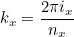#### Parameters

n (in)
global mode number
ix (out)
mode number in x-direction
iy (out)
mode number in y-direction
iz (out)
mode number in z-direction
teta_sym (out)
true if there is a cyclic computation

#### Example :

    EllipticProblem<LaplaceEquation<Dimension2> > var;;

// constructing the problem (mesh, finite element)
All_LinearSolver* solver;

// for a given mode number
int n = 23;
// decomposition in x, y, and z
int ix, iy, iz;
var.GetPeriodicModes(n, ix, iy, iz, teta_sym);

// phase (2 pi / ix)
Complex_wp kx, ky, kz;
var.GetPeriodicModes(n, kx, ky, kz);


### SetPeriodicCondition

#### Syntax

 void SetPeriodicCondition(Matrix& A )

This method applies periodic (or quasi-periodic) conditions to a given matrix. This treatment is relevant only for a strong formulation of periodic boundary conditions, where equationsreplaces the variational formulation for periodic degrees of freedom. In regular use, this method does not need to be called, since periodic boundary conditions are applied when AddMatrixWithBC is called.

### ApplyPeriodicCondition

#### Syntax

 void ApplyPeriodicCondition(Vector& x )

This method applies periodic (or quasi-periodic) conditions to the right hand side. This treatment is relevant only for a strong formulation of periodic boundary conditions, where equationsreplaces the variational formulation for periodic degrees of freedom. In regular use, this method does not need to be called, since periodic boundary conditions are applied when ComputeSolution is called.

### GetOrderAbsorbingCondition

#### Syntax

 int GetOrderAbsorbingCondition() const

This method returns the order of approximation for absorbing boundary conditions. For Helmholtz equations, it can be equal to 1 or 2. For other equations, only first-order absorbing boundary conditions are implemented.

#### Example :

    EllipticProblem<LaplaceEquation<Dimension2> > var;;

// constructing the problem (mesh, finite element)
All_LinearSolver* solver;

// order for ABCs ?
int r_abc = var.GetOrderAbsorbingCondition();


### GetNbEltPML

#### Syntax

 int GetNbEltPML() const

This method returns the number of elements inside the PMLs.

#### Example :

    EllipticProblem<LaplaceEquation<Dimension2> > var;;

// constructing the problem (mesh, finite element)
All_LinearSolver* solver;

// number of elements in PMLs (for current processor)
int n_pml = var.GetNbEltPML();


### GetNbGlobalEltPML

#### Syntax

 int GetNbGlobalEltPML() const

This method returns the global number of elements inside the PMLs. For a parallel computation, the method returns the sum of the number of elements (for all processors).

#### Example :

    EllipticProblem<LaplaceEquation<Dimension2> > var;;

// constructing the problem (mesh, finite element)
All_LinearSolver* solver;

// number of elements in PMLs (for all processors)
int n_pml = var.GetNbGlobalEltPML();


### MltMuIntegrationByParts

#### Syntax

 void MltMuIntegrationByParts(int ref, int ne , int num_loc , int k, Real_wp& coef) const

This method multiplies the coefficient by mu, which is a coefficient that appears in the boundary term when an integration by parts is performed. For instance, for Helmholtz equation, we have the term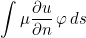#### Parameters

ref (in)
reference of the physical domain
ne (in)
element number
num_loc (in)
local face number
k (in)
mu (inout)
coefficient that will be multiplied by mu

#### Example :

    EllipticProblem<LaplaceEquation<Dimension2> > var;;

// constructing the problem (mesh, finite element)
All_LinearSolver* solver;

// for a given element
int ne = 14;
int ref = var.mesh.Element(ne).GetReference();
// local face of the element
int num_loc = 1, k = 14;

Real_wp coef(1);
// we want to compute coef = coef * mu
var.MltMuIntegrationByParts(ref, ne, num_loc, k, coef);


### GetMaximumVelocityPML

#### Syntax

 Real_wp GetMaximumVelocityPML() const

This method returns the maximum velocity in PMLs.

#### Example :

    EllipticProblem<LaplaceEquation<Dimension2> > var;;

// constructing the problem (mesh, finite element)
All_LinearSolver* solver;

// maximal velocity in PMLs ?
Real_wp vmax = var.GetMaximumVelocityPML();


### SetPhysicalIndexAtInfinity

#### Syntax

 void SetPhysicalIndexAtInfinity(const VectBool& RefUsed ) const

This method computes the physical index (rho and mu for Helmholtz equation) at infinity. This method is already called by ComputeMeshAndFiniteElement and does not need to be called in regular use.

### FindElementsInsidePML

#### Syntax

 void FindElementsInsidePML()

This method finds all the elements inside PMLs and marks them as PML elements. This method is already called by ComputeMeshAndFiniteElement and does not need to be called in regular use.

### EvaluateFunctionTauPML

#### Syntax

 void EvaluateFunctionTauPML(Real_wp dx , Real_wp sig , Real_wp a , Real_wp& zeta , Real_wp& zeta_p )

This method evaluates the damping function of the PML (usually a parabole).

#### Parameters

dx (in)
distance to the interface between the physical domain and PMLs
sig (in)
coefficient (damping is multiplied by this coefficient)
a (in)
thickness of the PML
zeta (out)
damping function
zeta_p (out)
primitive of zeta

#### Example :

    EllipticProblem<LaplaceEquation<Dimension2> > var;;

// constructing the problem (mesh, finite element)
All_LinearSolver* solver;

// damping function for a given distance ?
Real_wp zeta, zeta_p, thickness = 1.0;
var.EvaluateFunctionTauPML(dx, 1.0, thickness, zeta, zeta_p);


### GetDampingFactorPML

#### Syntax

 void GetDampingFactorPML(Mesh& mesh , int num_pml , int type_pml , R_N point , R_N& zeta , R_N& x_tilde )

This method evaluates the point after the complex variable change :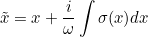that appears in PML layers. It computes also, the coefficient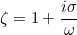that appears for derivatives with respect to x (y or z). zeta is a vector because it contains this factor for each coordinate x, y (and z in 3-D).

#### Parameters

mesh (in)
input mesh
num_pml (in)
PML number
type_pml (in)
type of PML
point (in)
point where zeta is computed
zeta (out)
damping factor
x_tilde (out)
point after complex change variable

#### Example :

    EllipticProblem<LaplaceEquation<Dimension2> > var;;

// constructing the problem (mesh, finite element)
All_LinearSolver* solver;

// damping factor on a given point
int ne = 12;
int num_pml = var.Element(ne).GetNumberPML();
int type_pml = var.Element(ne).GetTypePML();
R2 point(0.8, 2.4);
R2_Complex_wp zeta, x_tilde;
var.GetDampingFactorPML(var.mesh, num_pml, type_pml, point, zeta, x_tilde);


### GetDampingTauPML

#### Syntax

 void GetDampingTauPML(Mesh& mesh , int num_pml , int type_pml , R_N point , R_N& tau , R_N& tau_p )

This method evaluates the damping coefficient of the PML (in the three coordinates) and its primitive.

#### Parameters

mesh (in)
input mesh
num_pml (in)
PML number
type_pml (in)
type of PML
point (in)
point where zeta is computed
tau (out)
damping coefficient
tau_p (out)
primitive of damping coefficient

#### Example :

    EllipticProblem<LaplaceEquation<Dimension2> > var;;

// constructing the problem (mesh, finite element)
All_LinearSolver* solver;

// damping factor on a given point
int ne = 12;
int num_pml = var.Element(ne).GetNumberPML();
int type_pml = var.Element(ne).GetTypePML();
R2 point(0.8, 2.4);
R2_Complex_wp tau, tau_p;
var.GetDampingTauPML(var.mesh, num_pml, type_pml, point, tau, tau_p);


#### Syntax

 void AddMatrixImpedanceBoundary( Real_wp alpha, IVect ref_cond , int ref_target , GlobalGenericMatrix nat_mat , Matrix& mat_sp , int offset_row , int offset_col, ImpedanceFunction_Base& impedance, bool change_cols, bool change_rows, VarProblem& var)

This method adds a boundary integral :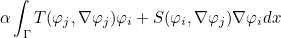to a sparse matrix. The local operators T and S are defined through the object impedance. The surface Γ over which the integral is computed consists of the facs such that the reference ref satisfy ref_cond(ref) = ref_target.

#### Parameters

alpha (in)
coefficient
ref_cond (in)
Surface gamma is selected such that ref_cond(ref) = ref_target
ref_target (in)
reference target
nat_mat (in)
mass, damping and stiffness coefficients
mat_sp (inout)
sparse matrix that will be modified
offset_row (in)
offset for row numbers
offset_col (in)
offset for column numbers
impedance (in)
class defining impedance operators T and S
change_cols (in)
column numbers are modified with NewColumnNumbers_Impedance
change_rows (in)
rows numbers are modified with NewRowNumbers_Impedance
var(in)
instance of VarProblem

#### Example :

    EllipticProblem<LaplaceEquation<Dimension2> > var;;

// constructing the problem (mesh, finite element)
All_LinearSolver* solver;

// definition of impedance coefficients
ImpedanceFunction_Base<Real_wp, Dimension2> impedance;
impedance.SetCoefficient(Real_wp(2), Real_wp(-1)); // case of constant scalar impedance
// i.e. T(phi, \nabla phi) = coef phi, S(phi, \nabla phi) = coef \nabla phi P
// P is the tangential projector to take into account only surface gradient

// initializing a sparse matrix
DistributedMatrix<Real_wp, Symmetric, ArrayRowSymSparse> mat_sp;
int N = var.GetNbDof(); mat_sp.Reallocate(N, N);

// selecting the surfaces of integration
IVect ref_cond(var.mesh.GetNbReferences()+1); ref_cond.Zero();
int ref_target = 1; ref_cond(2) = ref_target;

// calling the method to add the integral terms to the matrix
GlobalGenericMatrix<Real_wp> nat_mat;
mat_sp, 0, 0, impedance, false, false, var);


#### Syntax

 void AddBoundaryConditionTerms( Matrix& A, GlobalGenericMatrix nat_mat , int offset_row = 0, int offset_col = 0)

This method adds to the matrix the terms due to boundary conditions. On regular use, this method does not need to be called, since it is already called by AddMatrixWithBC.

#### Parameters

A (inout)
sparse matrix that will be modified
nat_mat (in)
mass, damping and stiffness coefficients
offset_row (optional)
offset for row numbers
offset_col (optional)
offset for column numbers

### InitCyclicDomain

#### Syntax

 void InitCyclicDomain()

This method initializes the computation of modes for cyclic or periodic domains. In regular use, this method does not need to be called since it is already called by ComputeMeshAndFiniteElement.

### ComputeQuasiPeriodicPhase

#### Syntax

 void ComputeQuasiPeriodicPhase
xo

This method computes the phase for quasi-periodic conditions.If you switch to another mode (for periodic/cyclic domains), this method needs to be called such that the quasi-periodic condition corresponds to the desired mode.

// The definition of the problem is constructed via EllipticProblem class
EllipticProblem<LaplaceEquation<Dimension2> > var;
var.InitIndices(100);
var.SetTypeEquation("LAPLACE"); // name of the equation, it can be used to use an equivalent formulation of the same equation

var.ComputeMeshAndFiniteElement("QUADRANGLE_LOBATTO"); // mesh and finite element are constructed
var.PerformOtherInitializations(); // other initializations
var.ComputeMassMatrix(); // computation of geometric quantities (such as jacobian matrices)
var.ComputeQuasiPeriodicPhase(); // for quasi-periodic conditions

// once var is constructed, you can call AddMatrixWithBC
GlobalGenericMatrix<Real_wp> nat_mat; // this object is used to set coefficients alpha, beta and gamma
// By default, alpha = beta = gamma = 1
Matrix<Real_wp, Symmetric, ArrayRowSymSparse> A;

// but you can change them
Real_wp alpha = 2.0, beta = 0.5, gamma = 0.25;
nat_mat.SetCoefMass(alpha);
nat_mat.SetCoefDamping(beta);
nat_mat.SetCoefStiffness(gamma);
// matrix is added, so you need to clear it if you do not want to keep
// previous non-zero entries
A.Clear();

// for an iterative matrix (the matrix is not necessary stored, use FemMatrixFreeClass)
FemMatrixFreeClass_Base<Real_wp>* Ah = var.GetNewIterativeMatrix(Real_wp(0));
delete Ah;


### AllocateTauPML

#### Syntax

 void AllocateTauPML()

This method allocates the arrays that will store damping terms for PMLs. In regular use, this method does not need to be called since it is already called by ComputeMassMatrix.

### GetPeriodicDofNumbers

#### Syntax

 void GetPeriodicDofNumbers(int i , int& k , int& j , int n = 0) const

This method retrieves the dofs numbers j, k of the periodic conditionfor the i-th periodic dof.

    EllipticProblem<LaplaceEquation<Dimension2> > var;;

// constructing the problem (mesh, finite element)
All_LinearSolver* solver;

// loops over periodic dofs
for (int i = 0; i < var.GetNbPeriodicDof(); i++)
{
// integers j and k such that u_k = u_j * phase
int j, k;
var.GetPeriodicDofNumbers(i, k, j);
}


### GetPeriodicPhase

#### Syntax

 void GetPeriodicPhase(int i , Complex_wp& phase ) const

This method retrieves the phase for the quasi-periodic condition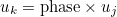for the i-th periodic dof.

    EllipticProblem<LaplaceEquation<Dimension2> > var;;

// constructing the problem (mesh, finite element)
All_LinearSolver* solver;

// loops over periodic dofs
for (int i = 0; i < var.GetNbPeriodicDof(); i++)
{
// integers j and k such that u_k = u_j * phase
int j, k;
var.GetPeriodicDofNumbers(i, k, j);

// phase
Complex_wp phase;
var.GetPeriodicPhase(i, phase);
}


### MltParamCondition

#### Syntax

 void MltParamCondition(int ref, int k, T& coef) const

This method multiplies a coefficient by the parameter given at a boundary condition.

    EllipticProblem<LaplaceEquation<Dimension2> > var;;

// constructing the problem (mesh, finite element)
All_LinearSolver* solver;

// reference of the physical surface
int ref = 2;

// then if you want to retrieve parameter 1 associated with this reference
// for instance if the data file contains ConditionReference = 2 IMPEDANCE 4.0 2.5
// parameter 0 is 4.0 and parameter 1 is 2.5
Real_wp coef(1);
var.MltParamCondition(2, 1, coef);


### GetImpedanceCoefficientABC

#### Syntax

 Complexe GetImpedanceCoefficientABC() const

This method returns the coefficient to modify the absorbing boundary condition. This coefficient is usually set by inserting a line ModifiedImpedance = 1.02 in the data file.

    EllipticProblem<HelmholtzEquation<Dimension2> > var;;

// constructing the problem (mesh, finite element)
All_LinearSolver* solver;

// coefficient to modify impedance for abc
Complex_wp coef = var.GetImpedanceCoefficientABC();


### GetTauPML

#### Syntax

 R_N GetTauPML(int num_elem , int k ) const Complexe GetTauPML(int num_elem , int k , int num_coor ) const

This method returns the value of the damping function for a quadrature point. This result is a vector because it contains the damping for each coordinate x, y (and z in 3-D). In the second syntax, we return the damping for a given coordinate.

#### Parameters

num_elem (in)
element number
k (in)
num_coor (in)
coordinate number

#### Example :

    EllipticProblem<LaplaceEquation<Dimension2> > var;;

// constructing the problem (mesh, finite element)
All_LinearSolver* solver;

// damping function on a given point
R2 tau; int num_elem = 12, k = 2;
tau = var.GetTauPML(num_elem, k);


### GetPrimitiveTauPML

#### Syntax

 Complexe GetPrimitiveTauPML(int num_elem , int k , int num_coor ) const

This method returns the value of the primitive of the damping function for a quadrature point.

#### Parameters

num_elem (in)
element number
k (in)
num_coor (in)
coordinate number

#### Example :

    EllipticProblem<LaplaceEquation<Dimension2> > var;;

// constructing the problem (mesh, finite element)
All_LinearSolver* solver;

// damping function on a given point
Real_wp tau_y; int num_elem = 12, k = 2, num_coor = 1;
tau_y = var.GetTauPML(num_elem, k, num_coor);

// primitive as well :
Real_wp tau_yp = var.GetPrimitiveTauPML(num_elem, k, num_coor);


### GetParamCondition

#### Syntax

 Complexe GetParamCondition(int ref, int k) const Vector& GetParamCondition() const

This method retrieves a single parameter associated with a reference number. You can also retrieves all parameters for all references.

    EllipticProblem<LaplaceEquation<Dimension2> > var;;

// constructing the problem (mesh, finite element)
All_LinearSolver* solver;

// reference of the physical surface
int ref = 2;

// then if you want to retrieve parameter 1 associated with this reference
// for instance if the data file contains ConditionReference = 2 IMPEDANCE 4.0 2.5
// parameter 0 is 4.0 and parameter 1 is 2.5
Real_wp coef = var.GetParamCondition(2, 1);

//  if you want to get all parameters
Vector<VectReral_wp> >& all_param = var.GetParamCondition();


### SetBoundaryConditionMesh

#### Syntax

 void SetBoundaryConditionMesh(int ref, int type) const

This method sets a boundary condition for a given reference. Usually the boundary conditions are given by inserting a line ConditionReference = 1 DIRICHLET in the data file.

    EllipticProblem<LaplaceEquation<Dimension2> > var;;

// if you want to set a boundary condition (not present in the data file)
var.SetBoundaryConditionMesh(1, BoundaryConditionEnum::LINE_NEUMANN);

// constructing the problem (mesh, finite element)
All_LinearSolver* solver;



#### Syntax

 void AddPeriodicConditionMesh(TinyVector ref, int type) const

This method sets a periodic boundary condition between two references. Usually the boundary conditions are given by inserting a line ConditionReference = 1 2 PERIODICITY in the data file.

    EllipticProblem<LaplaceEquation<Dimension2> > var;;

// if you want to set a periodic boundary condition (not present in the data file)

// constructing the problem (mesh, finite element)
All_LinearSolver* solver;



### GetNewImpedanceABC

#### Syntax

 ImpedanceFunction_Base* GetNewImpedanceABC(Complexe x) const

This method constructs an impedance object for absorbing boundary conditions. The argument given as parameters is used to retrieve an object dealing complex or real numbers.

    EllipticProblem<LaplaceEquation<Dimension2> > var;;

// constructing the problem (mesh, finite element)
All_LinearSolver* solver;

// impedance for absorbing boundary condition
ImpedanceFunction_Base<Real_wp, Dimension2>* imped = var.GetNewImpedanceABC();

// once used, delete the object
delete imped;


### GetNewImpedanceGeneric

#### Syntax

 ImpedanceFunction_Base* GetNewImpedanceGeneric(Complexe x) const

This method constructs an impedance object for absorbing boundary conditions. The argument given as parameters is used to retrieve an object dealing complex or real numbers.

    EllipticProblem<LaplaceEquation<Dimension2> > var;;

// constructing the problem (mesh, finite element)
All_LinearSolver* solver;

// impedance for Robin boundary condition
ImpedanceFunction_Base<Real_wp, Dimension2>* imped = var.GetNewImpedanceGeneric();

// once used, delete the object
delete imped;


### GetNewImpedanceHighConductivity

#### Syntax

 ImpedanceFunction_Base* GetNewImpedanceHighConductivity(Complexe x) const

This method constructs an impedance object for high conductivity boundary conditions. The argument given as parameters is used to retrieve an object dealing complex or real numbers.

    EllipticProblem<LaplaceEquation<Dimension2> > var;;

// constructing the problem (mesh, finite element)
All_LinearSolver* solver;

// impedance for high conductivity boundary condition
ImpedanceFunction_Base<Real_wp, Dimension2>* imped = var.GetNewImpedanceHighConductivity();

// once used, delete the object
delete imped;


### InvolveOnlyTangentialDofs

#### Syntax

 bool InvolveOnlyTangentialDofs() const

This method returns true if the boundary integrals are non-null only for degrees of freedom associated with the surface. Usually, it is the case. It can be false, for instance if the integral involves 3-D gradient of basis functions (and not surface gradients).

    EllipticProblem<LaplaceEquation<Dimension2> > var;;

// constructing the problem (mesh, finite element)
All_LinearSolver* solver;

// impedance for Robin boundary condition
ImpedanceFunction_Base<Real_wp, Dimension2>* imped = var.GetNewImpedanceGeneric();

bool only_tgt_dofs = imped->InvolveOnlyTangentialDofs();

// once used, delete the object
delete imped;


#### Syntax

This method returns true if the boundary integrals involve gradient (or curl/div) of basis functions. The aim is to save computational time when no gradients are needed.

    EllipticProblem<LaplaceEquation<Dimension2> > var;;

// constructing the problem (mesh, finite element)
All_LinearSolver* solver;

// impedance for Robin boundary condition
ImpedanceFunction_Base<Real_wp, Dimension2>* imped = var.GetNewImpedanceGeneric();

// once used, delete the object
delete imped;


### SetCoefficient

#### Syntax

 bool SetCoefficient(Complexe a, Complexe b)

This method sets the coefficients for the impedance. For scalar finite elements, these coefficients appear in the following term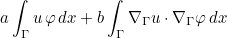EllipticProblem<LaplaceEquation<Dimension2> > var;;

// constructing the problem (mesh, finite element)
All_LinearSolver* solver;

// impedance for Robin boundary condition
ImpedanceFunction_Base<Real_wp, Dimension2>* imped = var.GetNewImpedanceGeneric();

// setting coefficients a and b in the expression a \int_\Gamma u \varphi dx + b \int_\Gamma \nabla_\Gamma u \cdot \nabla_\Gamma \varphi \, dx
Real_wp a = 2.0, b = 3.0;
imped->SetCoefficient(a, b);

// initializing a sparse matrix
DistributedMatrix<Real_wp, Symmetric, ArrayRowSymSparse> mat_sp;
int N = var.GetNbDof(); mat_sp.Reallocate(N, N);

// selecting the surfaces of integration
IVect ref_cond(var.mesh.GetNbReferences()+1); ref_cond.Zero();
int ref_target = 1; ref_cond(2) = ref_target;

// calling the method to add the integral terms to the matrix
GlobalGenericMatrix<Real_wp> nat_mat;
mat_sp, 0, 0, *imped, false, false, var);

// once used, delete the object
delete imped;


### GetCoefficient

#### Syntax

 bool GetCoefficient( int i, int num_elem, int num_loc, int k, int ref_domain, int ref SetPoints pts, SetMatrices mat)

This method returns the impedance coefficient at a given quadrature point.

#### Parameters

i (in)
face number
num_elem (in)
element number
num_loc (in)
local face number
k (in)
ref_domain (in)
reference for the physical domain
ref (in)
reference for the surface
pts (in)
mat (in)
jacobian matrices
    EllipticProblem<LaplaceEquation<Dimension2> > var;;

// constructing the problem (mesh, finite element)
All_LinearSolver* solver;

// impedance for Robin boundary condition
ImpedanceFunction_Base<Real_wp, Dimension2>* imped = var.GetNewImpedanceGeneric();

int i = 2; // edge number
int num_elem = var.mesh.BoundaryRef(i).numElement(0);
int num_loc = var.mesh.Element(num_elem).GetPositionBoundary(i);
int ref_domain = var.mesh.Element(num_elem).GetReference();
int ref = var.mesh.BoundaryRef(i).GetReference();

VectR2 s;
mesh.GetVerticesElement(num_elem, s);
const ElementReference_Dim<Dimension2>& Fb = var.GetReferenceElement(num_elem);
SetPoints<Dimension2> pts; SetMatrices<Dimension2> mat;
Fb.FjSurfaceElem(s, pts, mesh, num_elem, num_loc);
Fb.DFjSurfaceElem(s, pts, mat, mesh, num_elem, num_loc);

// evaluation of impedance
int k = 2; // local quadrature point number
GlobalGenericMatrix<Real_wp> nat_mat;
imped->EvaluateImpedancePhi(i, num_elem, i, num_loc, k, nat_mat, ref_domain, pts, mat);

// then if you want to get the coefficient
Real_wp coef = imped->GetCoefficient(i, num_elem, num_loc, k, ref_domain, ref, pts, mat);

// once used, delete the object
delete imped;


### EvaluateImpedancePhi

#### Syntax

 void EvaluateImpedancePhi( int i, int num_elem, int num_edge, int num_loc, int k, GlobalGenericMatrix nat_mat, int ref_domain, SetPoints pts, SetMatrices mat)

This method evaluates the impedance for a given quadrature point. In the method AddMatrixImpedanceBoundary, this method is called, such that the user can compute impedance coefficients (or operators). Then, the method ApplyImpedancePhi_H1 is called to apply the impedance against basis functions.

#### Parameters

i (in)
face number
num_elem (in)
element number
num_edge (in)
equal to i
num_loc (in)
local face number
k (in)
nat_mat (in)
mass, damping and stiffness coefficients
ref_domain (in)
reference for the physical domain
pts (in)
mat (in)
jacobian matrices
    EllipticProblem<LaplaceEquation<Dimension2> > var;;

// constructing the problem (mesh, finite element)
All_LinearSolver* solver;

// impedance for Robin boundary condition
ImpedanceFunction_Base<Real_wp, Dimension2>* imped = var.GetNewImpedanceGeneric();

int i = 2; // edge number
int num_elem = var.mesh.BoundaryRef(i).numElement(0);
int num_loc = var.mesh.Element(num_elem).GetPositionBoundary(i);
int ref_domain = var.mesh.Element(num_elem).GetReference();
int ref = var.mesh.BoundaryRef(i).GetReference();

VectR2 s;
mesh.GetVerticesElement(num_elem, s);
const ElementReference_Dim<Dimension2>& Fb = var.GetReferenceElement(num_elem);
SetPoints<Dimension2> pts; SetMatrices<Dimension2> mat;
Fb.FjSurfaceElem(s, pts, mesh, num_elem, num_loc);
Fb.DFjSurfaceElem(s, pts, mat, mesh, num_elem, num_loc);

// evaluation of impedance
int k = 2; // local quadrature point number
GlobalGenericMatrix<Real_wp> nat_mat;
imped->EvaluateImpedancePhi(i, num_elem, i, num_loc, k, nat_mat, ref_domain, pts, mat);

// then if you want to get the coefficient
Real_wp coef = imped->GetCoefficient(i, num_elem, num_loc, k, ref_domain, ref, pts, mat);

// once used, delete the object
delete imped;


#### Syntax

 void EvaluateImpedanceGrad( int i, int num_elem, int num_edge, int num_loc, int k, GlobalGenericMatrix nat_mat, int ref_domain, SetPoints pts, SetMatrices mat)

This method evaluates the impedance (for gradients) for a given quadrature point. In the method AddMatrixImpedanceBoundary, this method is called, such that the user can compute impedance coefficients (or operators). Then, the method ApplyImpedanceGrad is called to apply the impedance against gradient of basis functions.

#### Parameters

i (in)
face number
num_elem (in)
element number
num_edge (in)
equal to i
num_loc (in)
local face number
k (in)
nat_mat (in)
mass, damping and stiffness coefficients
ref_domain (in)
reference for the physical domain
pts (in)
mat (in)
jacobian matrices
    EllipticProblem<LaplaceEquation<Dimension2> > var;;

// constructing the problem (mesh, finite element)
All_LinearSolver* solver;

// impedance for Robin boundary condition
ImpedanceFunction_Base<Real_wp, Dimension2>* imped = var.GetNewImpedanceGeneric();

int i = 2; // edge number
int num_elem = var.mesh.BoundaryRef(i).numElement(0);
int num_loc = var.mesh.Element(num_elem).GetPositionBoundary(i);
int ref_domain = var.mesh.Element(num_elem).GetReference();
int ref = var.mesh.BoundaryRef(i).GetReference();

VectR2 s;
mesh.GetVerticesElement(num_elem, s);
const ElementReference_Dim<Dimension2>& Fb = var.GetReferenceElement(num_elem);
SetPoints<Dimension2> pts; SetMatrices<Dimension2> mat;
Fb.FjSurfaceElem(s, pts, mesh, num_elem, num_loc);
Fb.DFjSurfaceElem(s, pts, mat, mesh, num_elem, num_loc);

// evaluation of impedance
int k = 2; // local quadrature point number
GlobalGenericMatrix<Real_wp> nat_mat;
imped->EvaluateImpedancePhi(i, num_elem, i, num_loc, k, nat_mat, ref_domain, pts, mat);

// then if you want to get the coefficient
Real_wp coef = imped->GetCoefficient(i, num_elem, num_loc, k, ref_domain, ref, pts, mat);

imped->EvaluateImpedanceGrad(i, num_elem, i, num_loc, k, nat_mat, ref_domain, pts, mat);

// once used, delete the object
delete imped;


### ApplyImpedancePhi_H1

#### Syntax

 void ApplyImpedancePhi_H1( int m, int k, int offset, TinyVector phi, R2 grad_phi, Vector f_phi)

This method applies the impedance for a given quadrature point. In the method AddMatrixImpedanceBoundary, this method is called for the computation of the boundary integral.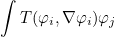where T is the impedance operator, i the row index and j the column index. This method can be overloaded to define your own impedance operator. This method involves only scalar basis functions (unknown number m is approximated with H1 Sobolev space).

#### Parameters

m (in)
unknown number (for rows)
j (in)
offset (in)
offset for the unknown m
phi (in)
value of basis function (row)
f_phi (in)
 // for your own impedance operator
class MyOperator : public ImpedanceFunction_Base<Real_wp, Dimension2>
{
void ApplyImpedancePhi_H1(int m, int j, int offset, const TinyVector<Real_wp, 1>& phi,

{
// for a diagonal operator :
Real_wp coef = 4.5; // impedance coefficient
f_phi(offset) = coef*phi(0);
}
};



#### Syntax

 void ApplyImpedanceGrad( int m, int k, int offset, TinyVector phi, R2 grad_phi, Vector f_phi)

This method applies the impedance for a given quadrature point. In the method AddMatrixImpedanceBoundary, this method is called for the computation of the boundary integral.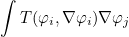where T is the impedance operator, i the row index and j the column index. This method can be overloaded to define your own impedance operator. This method involves only scalar basis functions (unknown number m is approximated with H1 Sobolev space).

#### Parameters

m (in)
unknown number (for rows)
j (in)
offset (in)
offset for the unknown m
phi (in)
value of basis function (row)
f_phi (in)
 // for your own impedance operator
class MyOperator : public ImpedanceFunction_Base<Real_wp, Dimension2>
{
void ApplyImpedancePhi_H1(int m, int j, int offset, const TinyVector<Real_wp, 1>& phi,

{
// for a diagonal operator :
f_phi.Zero();
Real_wp coef = 4.5; // impedance coefficient
f_phi(offset) = coef*phi(0);
}

void ApplyImpedanceGrad(int m, int j, int offset, const TinyVector<Real_wp, 1>& phi,

{
// if no gradient term, you can fill with zeros
f_phi.Zero();
}
};



### ApplyImpedancePhi_Hcurl

#### Syntax

 void ApplyImpedancePhi_Hcurl( int m, int k, int offset, TinyVector phi, TinyVector curl_phi, Vector f_phi)

This method applies the impedance for a given quadrature point. In the method AddMatrixImpedanceBoundary, this method is called for the computation of the boundary integral.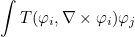where T is the impedance operator, i the row index and j the column index. This method can be overloaded to define your own impedance operator. This method involves only vectorial basis functions (unknown number m is approximated with H(curl) Sobolev space).

#### Parameters

m (in)
unknown number (for rows)
j (in)
offset (in)
offset for the unknown m
phi (in)
value of basis function (row)
curl_phi (in)
curl of basis function (row)
f_phi (in)
 // for your own impedance operator
class MyOperator : public ImpedanceFunction_Base<Real_wp, Dimension2>
{
void ApplyImpedancePhi_Hcurl(int m, int j, int offset, const TinyVector<Real_wp, 2>& phi,
const TinyVector<Real_wp, 1>& curl_phi, Vector<T>& f_phi)

{
// for a diagonal operator :
Real_wp coef = 4.5; // impedance coefficient
f_phi(offset) = coef*phi(0);
f_phi(offset+1) = coef*phi(1);
}
};



### ApplyImpedanceCurl

#### Syntax

 void ApplyImpedanceCurl( int m, int k, int offset, TinyVector phi, TinyVector curl_phi, Vector f_phi)

This method applies the impedance for a given quadrature point. In the method AddMatrixImpedanceBoundary, this method is called for the computation of the boundary integral.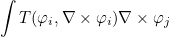where T is the impedance operator, i the row index and j the column index. This method can be overloaded to define your own impedance operator. This method involves only vectorial basis functions (unknown number m is approximated with H(curl) Sobolev space).

#### Parameters

m (in)
unknown number (for rows)
j (in)
offset (in)
offset for the unknown m
phi (in)
value of basis function (row)
curl_phi (in)
curl of basis function (row)
f_phi (in)
 // for your own impedance operator
class MyOperator : public ImpedanceFunction_Base<Real_wp, Dimension2>
{
void ApplyImpedancePhi_Hcurl(int m, int j, int offset, const TinyVector<Real_wp, 2>& phi,
const TinyVector<Real_wp, 1>& curl_phi, Vector<T>& f_phi)

{
// for a diagonal operator :
f_phi.Zero();
Real_wp coef = 4.5; // impedance coefficient
f_phi(offset) = coef*phi(0);
f_phi(offset+1) = coef*phi(1);
}

void ApplyImpedanceCurl(int m, int j, int offset, const TinyVector<Real_wp, 2>& phi,
const TinyVector<Real_wp, 1>& curl_phi, Vector<T>& f_phi)

{
// if no gradient term, you can fill with zeros
f_phi.Zero();
}
};



### ApplyImpedancePhi_Hdiv

#### Syntax

 void ApplyImpedancePhi_Hdiv( int m, int k, int offset, TinyVector phi, TinyVector div_phi, Vector f_phi)

This method applies the impedance for a given quadrature point. In the method AddMatrixImpedanceBoundary, this method is called for the computation of the boundary integral.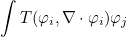where T is the impedance operator, i the row index and j the column index. This method can be overloaded to define your own impedance operator. This method involves only vectorial basis functions (unknown number m is approximated with H(div) Sobolev space).

#### Parameters

m (in)
unknown number (for rows)
j (in)
offset (in)
offset for the unknown m
phi (in)
value of basis function (row)
div_phi (in)
divergence of basis function (row)
f_phi (in)
 // for your own impedance operator
class MyOperator : public ImpedanceFunction_Base<Real_wp, Dimension2>
{
void ApplyImpedancePhi_Hdiv(int m, int j, int offset, const TinyVector<Real_wp, 2>& phi,
const TinyVector<Real_wp, 1>& div_phi, Vector<T>& f_phi)

{
// for a diagonal operator :
Real_wp coef = 4.5; // impedance coefficient
f_phi(offset) = coef*phi(0);
f_phi(offset+1) = coef*phi(1);
}
};



### ApplyImpedanceDiv

#### Syntax

 void ApplyImpedanceCurl( int m, int k, int offset, TinyVector phi, TinyVector div_phi, Vector f_phi)

This method applies the impedance for a given quadrature point. In the method AddMatrixImpedanceBoundary, this method is called for the computation of the boundary integral.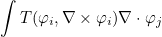where T is the impedance operator, i the row index and j the column index. This method can be overloaded to define your own impedance operator. This method involves only vectorial basis functions (unknown number m is approximated with H(div) Sobolev space).

#### Parameters

m (in)
unknown number (for rows)
j (in)
offset (in)
offset for the unknown m
phi (in)
value of basis function (row)
div_phi (in)
divergence of basis function (row)
f_phi (in)
 // for your own impedance operator
class MyOperator : public ImpedanceFunction_Base<Real_wp, Dimension2>
{
void ApplyImpedancePhi_Hdiv(int m, int j, int offset, const TinyVector<Real_wp, 2>& phi,
const TinyVector<Real_wp, 1>& div_phi, Vector<T>& f_phi)

{
// for a diagonal operator :
f_phi.Zero();
Real_wp coef = 4.5; // impedance coefficient
f_phi(offset) = coef*phi(0);
f_phi(offset+1) = coef*phi(1);
}

void ApplyImpedanceDiv(int m, int j, int offset, const TinyVector<Real_wp, 2>& phi,
const TinyVector<Real_wp, 1>& div_phi, Vector<T>& f_phi)

{
// if no gradient term, you can fill with zeros
f_phi.Zero();
}
};



### Constructor for TransparencySolver

#### Syntax

 TransparencySolver( EllipticProblem& var, All_LinearSolver& solver)

The constructor takes the considered problem to solve as argument. If you do not have an EllipticProblem instance, but rather a VarProblem_Base instance (if you are writing in a generic function), you can call GetNewTransparentSolver that will construct an object TransparencySolver and return a pointer of type TransparencySolver_Base.

int main()
{
EllipticProblem<HelmholtzEquation<Dimension2> > var;

// constructing the problem (mesh, finite element)
All_LinearSolver* solver;

GlobalGenericMatrix<Complex_wp> nat_mat; // this object is used to store mass, damping and stiffness coefficients
// to factorize the matrix (or prepare the computation if an iterative solver is selected)
solver->PerformFactorizationStep(nat_mat);

// right hand side
VectComplex_wp b(var.GetNbDof());
var.ComputeRightHandSide(b);

// and solve the linear system A x = b (with only first-order ABC)
VectComplex_wp x = b; solver->ComputeSolution(x);

// then you construct your transparent solver
TransparencySolver<HelmholtzEquation<Dimension2> > solver_transp(var, *solver);

// and you can iterate on the solution to obtain the exact solution
if (solver_transp.UseTransparentCondition())
{
solver_transp.Init();
VectComplex_wp source_rhs(x);
solver_transp.Solve(x, source_rhs);
}
}


### UseTransparentCondition

#### Syntax

 bool UseTransparentCondition() const

This method returns true if a transparent condition has been set.

int main()
{
EllipticProblem<HelmholtzEquation<Dimension2> > var;

// constructing the problem (mesh, finite element)
All_LinearSolver* solver;

GlobalGenericMatrix<Complex_wp> nat_mat; // this object is used to store mass, damping and stiffness coefficients
// to factorize the matrix (or prepare the computation if an iterative solver is selected)
solver->PerformFactorizationStep(nat_mat);

// right hand side
VectComplex_wp b(var.GetNbDof());
var.ComputeRightHandSide(b);

// and solve the linear system A x = b (with only first-order ABC)
VectComplex_wp x = b; solver->ComputeSolution(x);

// then you construct your transparent solver
TransparencySolver<HelmholtzEquation<Dimension2> > solver_transp(var, *solver);

// and you can iterate on the solution to obtain the exact solution
if (solver_transp.UseTransparentCondition())
{
solver_transp.Init();
VectComplex_wp source_rhs(x);
solver_transp.Solve(x, source_rhs);
}
}


### Solve

#### Syntax

 void Solve(Vector& x_sol, Vector& b_src) const

This method fills the vector x_sol to contain the solution with a transparent condition. The input vector b_src contains the solution with a first-order absorbing boundary condition.

int main()
{
EllipticProblem<HelmholtzEquation<Dimension2> > var;

// constructing the problem (mesh, finite element)
All_LinearSolver* solver;

GlobalGenericMatrix<Complex_wp> nat_mat; // this object is used to store mass, damping and stiffness coefficients
// to factorize the matrix (or prepare the computation if an iterative solver is selected)
solver->PerformFactorizationStep(nat_mat);

// right hand side
VectComplex_wp b(var.GetNbDof());
var.ComputeRightHandSide(b);

// and solve the linear system A x = b (with only first-order ABC)
VectComplex_wp x = b; solver->ComputeSolution(x);

// then you construct your transparent solver
TransparencySolver<HelmholtzEquation<Dimension2> > solver_transp(var, *solver);

// and you can iterate on the solution to obtain the exact solution
if (solver_transp.UseTransparentCondition())
{
solver_transp.Init();
VectComplex_wp source_rhs(x);
solver_transp.Solve(x, source_rhs);
}
}


### Init

#### Syntax

 void Init()

This method initializes the computation of the transparent condition. It will compute quadrature points and weights for the two surfaces (absorbing surface and intermediary surface).

int main()
{
EllipticProblem<HelmholtzEquation<Dimension2> > var;

// constructing the problem (mesh, finite element)
All_LinearSolver* solver;

GlobalGenericMatrix<Complex_wp> nat_mat; // this object is used to store mass, damping and stiffness coefficients
// to factorize the matrix (or prepare the computation if an iterative solver is selected)
solver->PerformFactorizationStep(nat_mat);

// right hand side
VectComplex_wp b(var.GetNbDof());
var.ComputeRightHandSide(b);

// and solve the linear system A x = b (with only first-order ABC)
VectComplex_wp x = b; solver->ComputeSolution(x);

// then you construct your transparent solver
TransparencySolver<HelmholtzEquation<Dimension2> > solver_transp(var, *solver);

// and you can iterate on the solution to obtain the exact solution
if (solver_transp.UseTransparentCondition())
{
solver_transp.Init();
VectComplex_wp source_rhs(x);
solver_transp.Solve(x, source_rhs);
}
}


### ComputeSolution

#### Syntax

 void ComputeSolution(Vector& rhs, Vector& sol)

This method computes the solution of the sparse finite element matrix As sol = rhs.

int main()
{
EllipticProblem<HelmholtzEquation<Dimension2> > var;

// constructing the problem (mesh, finite element)
All_LinearSolver* solver;

GlobalGenericMatrix<Complex_wp> nat_mat; // this object is used to store mass, damping and stiffness coefficients
// to factorize the matrix (or prepare the computation if an iterative solver is selected)
solver->PerformFactorizationStep(nat_mat);

// right hand side
VectComplex_wp b(var.GetNbDof());
var.ComputeRightHandSide(b);

// then you construct your transparent solver
TransparencySolver<HelmholtzEquation<Dimension2> > solver_transp(var, *solver);

// we solve the linear system A_s x = b (with only first-order ABC)
VectComplex_wp x = b; solver_transp.ComputeSolution(b, x);

// and you can iterate on the solution to obtain the exact solution
if (solver_transp.UseTransparentCondition())
{
solver_transp.Init();
VectComplex_wp source_rhs(x);
solver_transp.Solve(x, source_rhs);
}

}


### ComputeGreenKernel

#### Syntax

 void ComputeGreenKernel(const R3& x, const R3& y, const Real_wpp& k, Complex_wp& phi, R3_Complex_wp& grad_phi, Matrix3_3sym_Complex_wp& hessian_phi) void ComputeGreenKernel(const R3& x, const R3& y, const Real_wpp& k, Complex_wp& phi, R3_Complex_wp& grad_phi)

This static method computes the Green kernel (for wave equation), its gradient (with respect to y) and hessian matrix. You can also compute only the kernel and its gradient. This Green's kernel is equal to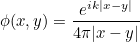#### Parameters

x (in)
point
y (in)
point
k (in)
wave number
phi (out)
Green's kernel phi(x, y)
hessian_phi (out, optional)
hessian of Green's kernel
int main()
{
// you choose two points
R3 x(0.1, 0.5, 0.3), y(0.2, 2.0, 0.8);

// a wave number
Real_wp k = 0.3;

// Green's kernel and gradient for these values
Complex_wp phi;
TransparencySolver_Base::ComputeGreenKernel(x, y, k, phi, grad_phi, hessian_phi);
}


### ComputeSurfaceGammaAndAbsorbing

#### Syntax

 void ComputeSurfaceGammaAndAbsorbing(int ref_abc, int ref_gamma, IVect& offset_abc_proc)

This internal method computes the quadrature points for the intermediary surface (denoted Gamma) and for the absorbing surface (denoted sigma). ref_abc is the boundary condition associated with the absorbing surface (usually equal to BoundaryConditionEnum::LINE_ABSORBING. ref_gamma is the body number for the intermediary surface (all references such that the body number is equal to ref_gamma will belong to this intermediary surface). The output argument offset_abc_proc stores the offsets for quadrature points of the absorbing surface. On regular use, this methods does not need to be called, since the method Init will call it automatically.

### ComputeRightHandSide

#### Syntax

 void ComputeRightHandSide(const VectComplex_wp& x_sol, VectComplex_wp& g_source)

This internal method computes the matrix-vector product g_source = Ad x_sol where Ad is introduced in the section devoted to transparent conditions. On regular use, this method does not need to be called, since the method Solve will call it automatically.

### ComputeIntegralRepresentation

#### Syntax

 void ComputeIntegralRepresentation( const VectComplex_wp& trace_En, const VectComplex_wp& trace_Hn, const MeshInterpolation& mesh_gamma, const R_N& x, const R_N& n, TinyVector& En, TinyVector& Hn)

This method computes the value of E x n and H x n on a given point from values on the quadrature points of the intermediary surface Gamma (by using an integral representation). On regular use, this method does not need to be called, since the method Solve will call it automatically. This method is virtual such that it is overloaded for each equation where it is implemented.

#### Parameters

trace_En (in)
values of E x n on quadrature points of Gamma
trace_Hn (in)
values of H x n on quadrature points of Gamma
mesh_gamma (in)
surface mesh Gamma
x (in)
point where E x n will be evaluated
n (in)
outgoing normale associated with the point x
En (out)
evaluation of E x n at x (with the given normale)
Hn (out)
evaluation of H x n at x (with the given normale)

### ComputeAndStoreEnPot

#### Syntax

 void ComputeAndStoreEnPot(const VectComplex_wp& trace_En, const VectComplex_wp& trace_Hn, const R_N& x, const R_N& n, Vector& En, Vector& Hn, int k)

This method computes the value of E x n and H x n on a given point from values on the quadrature points of the intermediary surface Gamma (by using an integral representation). On regular use, this method does not need to be called, since the method Solve will call it automatically. This method is virtual such that it is overloaded for each equation where it is implemented. This method actually calls ComputeIntegralRepresentation and inserts the results on the global vectors En and Hn .

#### Parameters

trace_En (in)
values of E x n on quadrature points of Gamma
trace_Hn (in)
values of H x n on quadrature points of Gamma
x (in)
point where E x n will be evaluated
n (in)
outgoing normale associated with the point x
EnStore (inout)
evaluation of E x n for all points of Sigma
HnStore (out)
evaluation of H x n for all points of Sigma
k (out)
number of the point x (it corresponds to the position where E x n and H x n will be inserted in EnStore and HnStore)

### GetSource

#### Syntax

 void GetSource( const VectComplex_wp& trace_En, const VectComplex_wp& trace_Hn, int n, Real_wp k, const R_N& ptX, const R_N& normaleX, Vector& g_source, int k_loc)

This method computes the source term g, that appears in the matrix Ad. This matrix will be equal to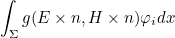On regular use, this method does not need to be called, since the method Solve will call it automatically. This method is virtual such that it is overloaded for each equation where it is implemented.

#### Parameters

trace_En (in)
values of E x n on quadrature points of Sigma
trace_Hn (in)
values of H x n on quadrature points of Sigma
n (in)
global point number where g needs to be evaluated
k (in)
wave number at infinity
ptX (in)
point where g needs to be evaluated
normaleX (out)
outgoing normale associated with ptX
g_source (inout)
source term that will be modified for the considered quadrature point
j (in)

### RcsToBeComputed

#### Syntax

 bool RcsToBeComputed()

This method will return true if the computation of a radar cross section is asked by the user.

int main()
{
EllipticProblem<HelmholtzEquation<Dimension2> > var;

// constructing the problem (mesh, finite element)
All_LinearSolver* solver;

// an object VarComputationRCS is contained in var :
VarComputationRCS<HelmholtzEquation<Dimension2> >& var_rcs = var.output_rcs_param;

if (var_rcs.RcsToBeComputed())
cout << "RCS has to be computed" << endl;
}


### GetNbAngles

#### Syntax

 int GetNbAngles()

This method returns the number of angles required for the radar cross section. This number is usually set by inserting a line AngleRCS = 0.0 180.0 181 in the data file.

int main()
{
EllipticProblem<HelmholtzEquation<Dimension2> > var;

// constructing the problem (mesh, finite element)
All_LinearSolver* solver;

// an object VarComputationRCS is contained in var :
VarComputationRCS<HelmholtzEquation<Dimension2> >& var_rcs = var.output_rcs_param;

if (var_rcs.RcsToBeComputed())
cout << "Number of angles for the RCS = " << var_rcs.GetNbAngles() << endl;
}


### GetNbPointsOutside

#### Syntax

 int GetNbPointsOutside()

This method returns the number of points outside the computational domain for which the user wants to evaluate the solution. For these points, the field will be computed with an integral representation. This number is usually set by inserting a line SismoOutsidePoints = pts.txt diffrac.dat total.dat 0.1 in the data file.

int main()
{
EllipticProblem<HelmholtzEquation<Dimension2> > var;

// constructing the problem (mesh, finite element)
All_LinearSolver* solver;

// an object VarComputationRCS is contained in var :
VarComputationRCS<HelmholtzEquation<Dimension2> >& var_rcs = var.output_rcs_param;

if (var_rcs.RcsToBeComputed())
cout << "Number of points outside = " << var_rcs.GetNbPointsOutside() << endl;
}


### GetRcsType

#### Syntax

 int GetRcsType()

This method returns the type of radar cross section to be computed. This type is usually set by inserting a line ParametersRCS = YES 1 AUTO BISTATIC in the data file. The integer can be equal to VarComputationRCS_Base<Dimension>::MONOSTATIC_RCS or VarComputationRCS_Base<Dimension>::BISTATIC_RCS.

int main()
{
EllipticProblem<HelmholtzEquation<Dimension2> > var;

// constructing the problem (mesh, finite element)
All_LinearSolver* solver;

// an object VarComputationRCS is contained in var :
VarComputationRCS<HelmholtzEquation<Dimension2> >& var_rcs = var.output_rcs_param;

if (var_rcs.RcsToBeComputed())
if (var_rcs.GetRcsType() == var_rcs.MONOSTATIC_RCS)
cout << "computation of a monostatic radar cross section" << endl;
}


### GetOutsidePoint

#### Syntax

 const VectR_N& GetOutsidePoint() const R_N& GetOutsidePoint(int i)

This method returns the points outside the computational domain for which the user wants to evaluate the solution. For these points, the field will be computed with an integral representation. These points are usually set by inserting a line SismoOutsidePoints = pts.txt diffrac.dat total.dat 0.1 in the data file.

int main()
{
EllipticProblem<HelmholtzEquation<Dimension2> > var;

// constructing the problem (mesh, finite element)
All_LinearSolver* solver;

// an object VarComputationRCS is contained in var :
VarComputationRCS<HelmholtzEquation<Dimension2> >& var_rcs = var.output_rcs_param;

// to retrieve all the points outside the computational domain
const VectR2& pts_outside = var_rcs.GetOutsidePoint();

// to retrieve a single point
int num_pt = 2;
const R2& pt = var_rcs.GetOutsidePoint(num_pt);
}


### SetOutsidePoints

#### Syntax

 void SetOutsidePoints(const VectR_N& pts)

This method sets the points outside the computational domain for which the user wants to evaluate the solution. For these points, the field will be computed with an integral representation. These points are usually set by inserting a line SismoOutsidePoints = pts.txt diffrac.dat total.dat 0.1 in the data file.

int main()
{
EllipticProblem<HelmholtzEquation<Dimension2> > var;

// constructing the problem (mesh, finite element)
All_LinearSolver* solver;

// an object VarComputationRCS is contained in var :
VarComputationRCS<HelmholtzEquation<Dimension2> >& var_rcs = var.output_rcs_param;

// if you want to change the list of outside points
VectR2 pts(2);
pts(0).Init(5.0, 2.0);
pts(1).Init(8.0, 1.0);

var_rcs.SetOutsidePoints(pts);
}


### GetInterpolationMesh

#### Syntax

 MeshInterpolationFEM& GetInterpolationMesh()

This method returns the mesh of the surface used to compute the radar cross section. This mesh contains quadrature points and normales.

int main()
{
EllipticProblem<HelmholtzEquation<Dimension2> > var;

// constructing the problem (mesh, finite element)
All_LinearSolver* solver;

// an object VarComputationRCS is contained in var :
VarComputationRCS<HelmholtzEquation<Dimension2> >& var_rcs = var.output_rcs_param;

var_rcs.InitComputationRCS(); // actually useless, since already called above in ConstructAll

const MeshInterpolationFEM<Dimension2>& surf_mesh = var_rcs.GetInterpolationMesh();
}


### SetTimeStep

#### Syntax

 void SetTimeStep(Real_wp dt)

This method sets the time step used for unsteady simulations.

int main()
{
EllipticProblem<HelmholtzEquation<Dimension2> > var;

// constructing the problem (mesh, finite element)
All_LinearSolver* solver;

// an object VarComputationRCS is contained in var :
VarComputationRCS<HelmholtzEquation<Dimension2> >& var_rcs = var.output_rcs_param;

var_rcs.SetTimeStep(0.1);
var_rcs.InitComputationRCS();
}


### InitComputationRCS

#### Syntax

 void InitComputationRCS(bool assemble = false)

This method initializes the computation of the radar cross section. It mainly computes the quadrature points and normales for the surface used to compute the integrals.

int main()
{
EllipticProblem<HelmholtzEquation<Dimension2> > var;

// constructing the problem (mesh, finite element)
All_LinearSolver* solver;

// an object VarComputationRCS is contained in var :
VarComputationRCS<HelmholtzEquation<Dimension2> >& var_rcs = var.output_rcs_param;

var_rcs.InitComputationRCS(); // actually useless, since already called above in ConstructAll
}


### ComputeRCS

#### Syntax

 void ComputeRCS(VectComplex_wp x_sol)

This method computes the radar cross section for the solution given as argument. The results are written in the file given in the datafile (FileRCS = Rcs.dat).

int main()
{
EllipticProblem<HelmholtzEquation<Dimension2> > var;

// constructing the problem (mesh, finite element)
All_LinearSolver* solver;

// an object VarComputationRCS is contained in var :
VarComputationRCS<HelmholtzEquation<Dimension2> >& var_rcs = var.output_rcs_param;

var_rcs.InitComputationRCS(); // actually useless, since already called above in ConstructAll

GlobalGenericMatrix<Complex_wp> nat_mat; // this object is used to store mass, damping and stiffness coefficients
// to factorize the matrix (or prepare the computation if an iterative solver is selected)
solver->PerformFactorizationStep(nat_mat);

// right hand side
VectComplex_wp b(var.GetNbDof());
var.ComputeRightHandSide(b);

// and solve the linear system A x = b (with only first-order ABC)
VectComplex_wp x = b; solver->ComputeSolution(x);

// computation of the radar cross section for this solution
var_rcs.ComputeRCS(x);
}


### ComputeIntegralRepresentation

#### Syntax

 void ComputeIntegralRepresentation( const VectComplex_wp& trace_En, const VectComplex_wp& trace_Hn, const MeshInterpolationFEM& mesh, R_N ptX, VectComplex_wp& u)

This method computes the solution on a point outside the computational domain with an integral representation.

#### Parameters

trace_En (in)
values of E x n on quadrature points on the surface of integration
trace_Hn (in)
values of H x n on quadrature points on the surface of integration
mesh (in)
mesh of the surface
ptX (in)
point where the solution needs to be evaluated
u (out)
result
int main()
{
EllipticProblem<HelmholtzEquation<Dimension2> > var;

// constructing the problem (mesh, finite element)
All_LinearSolver* solver;

// an object VarComputationRCS is contained in var :
VarComputationRCS<HelmholtzEquation<Dimension2> >& var_rcs = var.output_rcs_param;

var_rcs.InitComputationRCS(); // actually useless, since already called above in ConstructAll

GlobalGenericMatrix<Complex_wp> nat_mat; // this object is used to store mass, damping and stiffness coefficients
// to factorize the matrix (or prepare the computation if an iterative solver is selected)
solver->PerformFactorizationStep(nat_mat);

// right hand side
VectComplex_wp b(var.GetNbDof());
var.ComputeRightHandSide(b);

// and solve the linear system A x = b (with only first-order ABC)
VectComplex_wp x = b; solver->ComputeSolution(x);

// computation of E x n and H x n on the surface of integration
VectComplex_wp traceEn, traceHn;
var.ComputeEnHnOnBoundary(var_rcs.GetInterpolationMesh(), x, traceEn, traceHn, false);

// if you want to know the solution on a point outside the computational domain
R2 ptX(3.0, 0.8); VectComplex_wp u(1);
var_rcs.ComputeIntegralRepresentation(traceEn, traceHn, var_rcs.GetInterpolationMesh(), ptX, u);
}